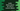# Binary search implementation in Kotlin for a list of custom objects## Binary search implementation in Kotlin for a list of custom objects:

Kotlin Collection class comes with a binary search method that can be used to search in a collection like list. We can also use this method to search in a list of custom objects. In this post, we will learn how to implement binary search in a list of custom objects.

This method is defined as below:

``ist<T>.binarySearch(element: T, comparator: Comparator<in T>, fromIndex: Int = 0, toIndex: Int = size): Int``

Here, element - It is the element to search in the list. comparator - It is the comparator and the list is expected to be sorted according to the specified comparator. fromIndex and toIndex are indices where to search in the list. By default, fromIndex is equal to 0 and toIndex is equal to the size of the list.

The return value:

• is the index of the element, if it is found in the list in the given range
• Otherwise, it returns inverted insertion point (-insertion point - 1). Insertion point is the index at which the element should be present so that the list remains sorted as per the comparator.

### Example to use binary search in Kotlin list with custom objects:

Let’s take a look at the below example program:

``````package com.company

data class Student(val name: String, val age: Int)

class CompareStudents {
companion object : Comparator<Student> {
override fun compare(o1: Student, o2: Student): Int {
return (o1.age - o2.age)
}
}
}

fun main() {
val studentList = listOf(
Student("Alex", 20),
Student("Bob", 22),
Student("Chandler", 25),
Student("Dave", 27),
Student("Daisy", 28)
)
val studentToFind = Student("Brian", 22)

println(studentList.binarySearch(studentToFind, CompareStudents))
}``````

Here,

• Student is a data class to hold the name and age of a student.
• CompareStudents class is used as comparator. We can use this comparator to search for a Student in the list if any other Student exists with equal age as the provided student.

If you run this above program, it will print:

``1``

### Searching in a range:

We can also pass the start and end index to search in the list:

``println(studentList.binarySearch(studentToFind, CompareStudents,0, 5))``

It will search in between 0 to 5.